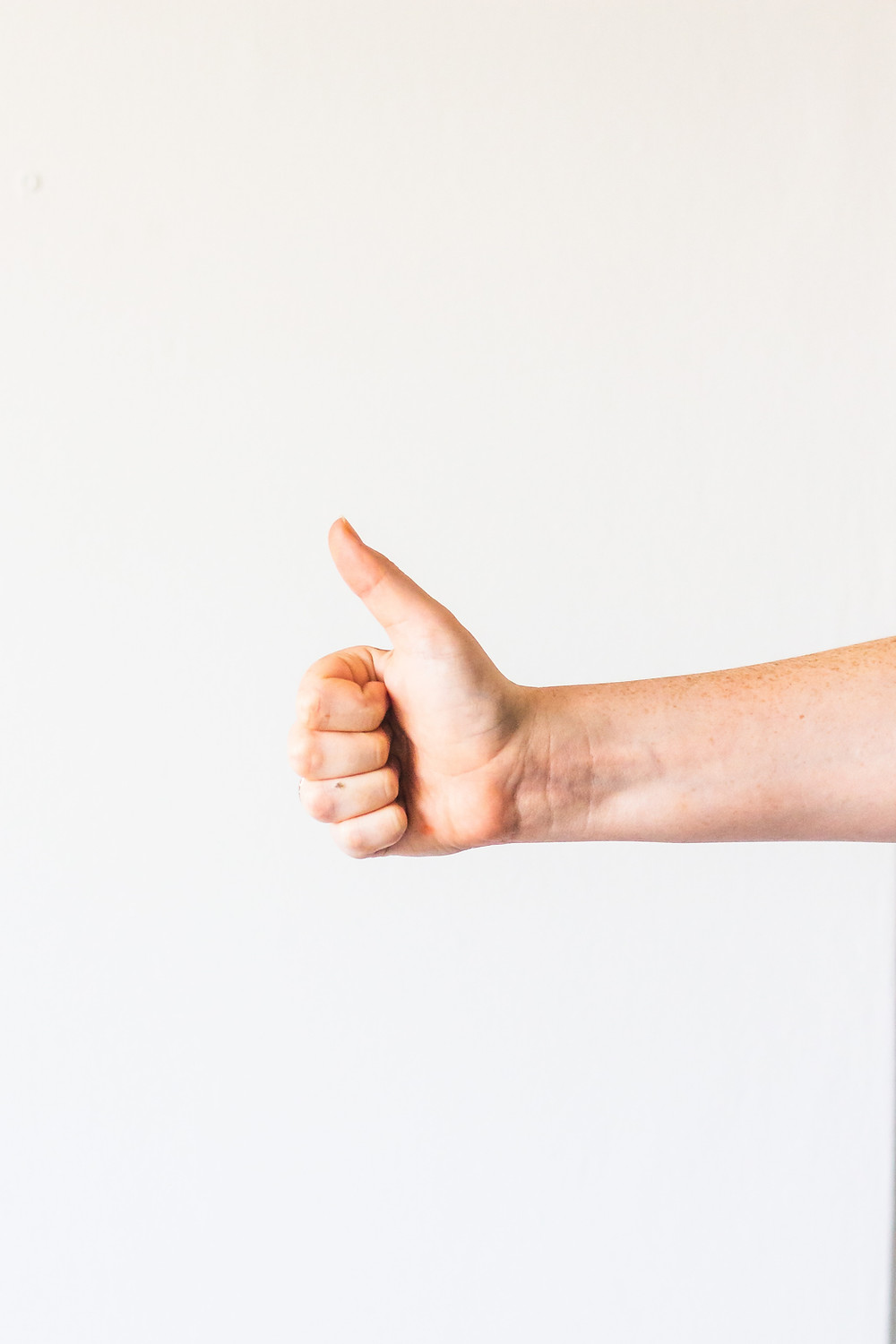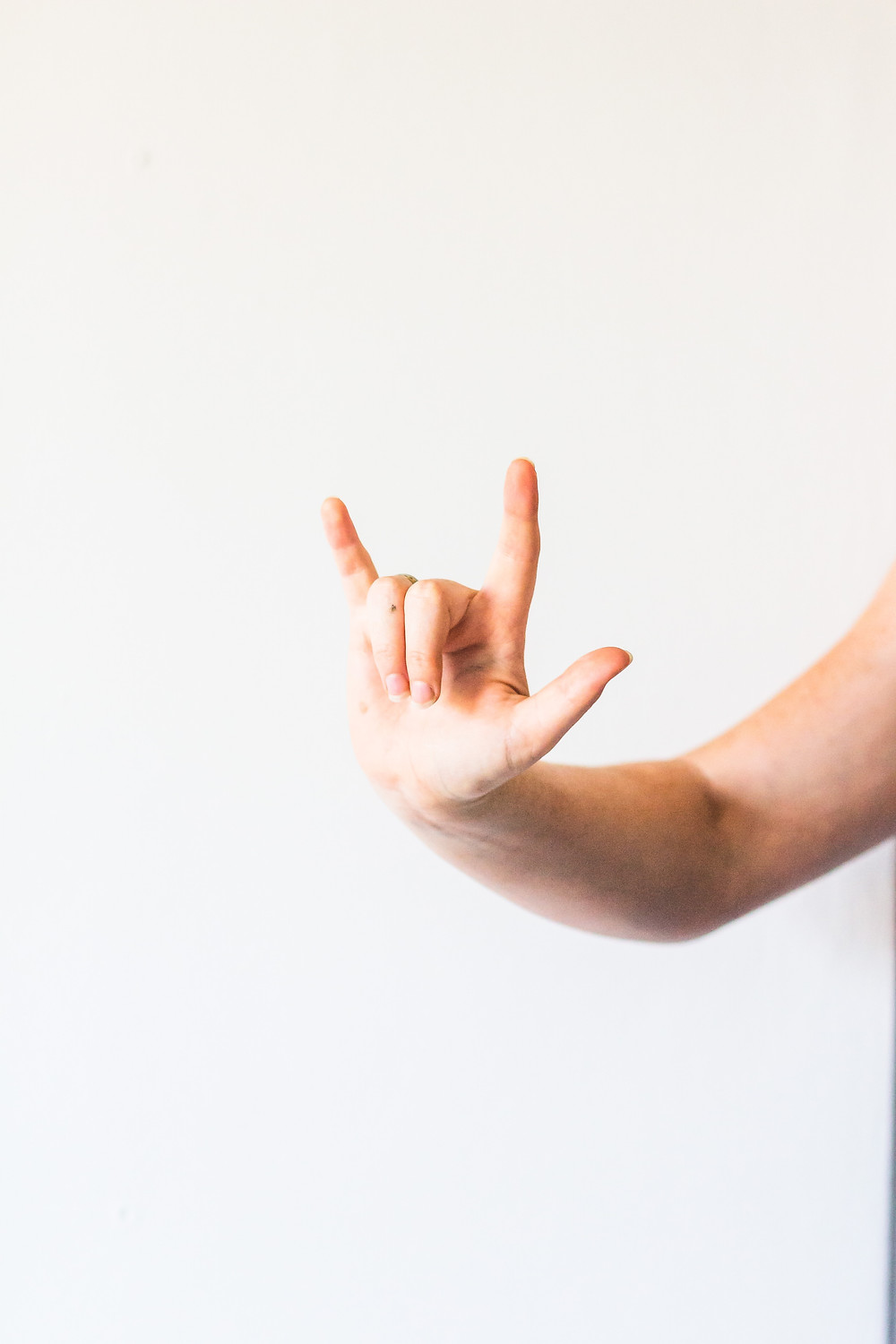Search
•Alibek Jakupov

# Azure Custom Vision: analyze multiple objects simultaneously using Tensorflow

Updated: Nov 19, 2021In this short article we are going to classify multiple objects simultaneosly in real time using Python and Tensorflow.

## When may this be useful?

Imagine you analyze gestures in real time. You've trained your Custom Vision model to classify different gestures and to improve the model quality you limited the region of interest in this way.Naturally, as we have two hands, we would like to analyze two hands simultaneously using the same model. Here are the steps to set this kind of solution up and running.

## Why on local?

When you do the analysis in real time you need to analyze each frame, each milisecond. Consequently, when you call API, at each iteration you will need to open the URL connection, prepare image, send the HTTP request, get HTTP response, and finally, parse the JSON output. It's not too long in principle, but it is definetely much longer than a milisecond, so you can hardly call it "real-time". We will see how to do it on local, just within a couple of miliseconds.

First of all we need to train the model. At this stage there should be no problem. Simply upload your images, tag them and launch the training. Important: before starting your project be sure to make it ‘exportable’, i.e. select compact option.This step should not be too complicated. After the training ends (usually it’s a matter of few seconds) go to Performance tab and click on Export button. In the dialog menu choose Tensor Flow (Android) and download it. The key word for us is Tensor Flow and not Android, as we are going to use it in our Python application.## Create a Hand Class

To make your code more 'clean' and readable, let us create a separate class containing all the hand parameters, like position and color. We'he also added all the image preprocessing and classification steps as the class methods.

```# coding utf-8
import cv2
import tensorflow as tf
import numpy as np

class Hand:

# BGR colors
BLACK = (0, 0, 0)
WHITE = (255, 255, 255)
BLUE = (255, 0, 0)
GREEN = (0, 255, 0)
RED = (0, 0, 255)
# Output text parameters
FONT = cv2.FONT_HERSHEY_SIMPLEX
FONT_SCALE = 1
LINE_TYPE = 1
# list of classes
LABELS = ['Vicky', 'Rock']
INPUT_NODE = 'Placeholder:0'

def __init__(self, frame, upX, upY, w, h, prob_tensor, sess, network_input_size):
self.frame = frame
self.upX = upX
self.upY = upY
self.w = w
self.h = h
self.lowX = upX + w
self.lowY = upY + h
self.network_input_size = network_input_size
self.prob_tensor = prob_tensor
self.sess = sess
self.image = self.frame[self.upY: self.lowY, self.upX: self.lowX]

def resize_down_to_1600_max_dim(self, image):
"""Change oversized image dimensions using Linear Interpolation

Arguments:
image {OpenCV} -- OpenCV image

Returns:
OpenCV -- resized or initial image
"""
h, w = image.shape[:2]
if (h < 1600 and w < 1600):
return image

new_size = (1600 * w // h, 1600) if (h > w) else (1600, 1600 * h // w)
return cv2.resize(image, new_size, interpolation=cv2.INTER_LINEAR)

def crop_center(self, img, cropx, cropy):
"""Extract a middle part of an image

Arguments:
img {OpenCv} -- OpenCV image to be cropped
cropx {[type]} -- width of the cropped region
cropy {[type]} -- height of the cropped region

Returns:
[OpenCV] -- cropped image
"""
h, w = img.shape[:2]
startx = w//2-(cropx//2)
starty = h//2-(cropy//2)
return img[starty:starty+cropy, startx:startx+cropx]

def resize_to_256_square(self, image):
"""Resize an image using the Linear Interpolation

Arguments:
image {OpenCV} -- OpenCV image

Returns:
OpenCV -- resized image
"""
h, w = image.shape[:2]
return cv2.resize(image, (256, 256), interpolation=cv2.INTER_LINEAR)

"""Prepare image for Tensorflow model

Args:
image (OpenCV): input image
network_input_size (int): tensorflow model input size

Returns:
OpenCV: preprocessed image, ready to be passed to the model
"""

# Crop the center for the specified network_input_Size
augmented_image = cv2.resize(
image, (self.network_input_size, self.network_input_size), interpolation=cv2.INTER_LINEAR)
return augmented_image

def get_color(self):

predictions = self.sess.run(

# get the highest probability label
highest_probability_index = np.argmax(predictions)
self.predicted_tag = self.LABELS[highest_probability_index]

if self.predicted_tag == 'Vicky':
frame_color = self.GREEN
elif self.predicted_tag == 'Rock':
frame_color = self.RED
else:
frame_color = self.RED

return frame_color```

The logic is pretty simple : we pass the coordinates to the class constructor and predict its class inside, and, according to the predicted class set the frame color.

## Get Left and Right hands

At this step we simple define the hand's position and pass them to the class constructor as well as a probability tensor, that will be used for predictions.

```def get_left_hand(frame, prob_tensor, sess, network_input_size):
"""Crop left part corresponding to the position of the left hand

Args:
image (OpenCV): input frame

Returns:
Hand: instance of the Hand class
"""
upX, upY, w, h = 50, 50, 150, 350
return Hand(frame, upX, upY, w, h, prob_tensor, sess, network_input_size)

def get_right_hand(frame, prob_tensor, sess, network_input_size):
"""Crop left part corresponding to the position of the right hand

Args:
image (OpenCV): input frame

Returns:
Hand: instance of the Hand class
"""
_, frame_width = frame.shape[:2]
w, h = 150, 350
upX, upY = frame_width-w-50, 50
return Hand(frame, upX, upY, w, h, prob_tensor, sess, network_input_size)```

The network input size is obtained in the main function. Our image will be resized to fit this input network size.

Do predictions in real time

We are now ready to run the model and classify our hands

```with tf.compat.v1.Session() as sess:
prob_tensor = sess.graph.get_tensor_by_name(output_layer)

# Get the input size of the model
input_tensor_shape = sess.graph.get_tensor_by_name(
input_node).shape.as_list()
network_input_size = input_tensor_shape

while(video_capture.isOpened()):
# read video frame by frame

frame = cv2.flip(frame, 1)
frame_counter += 1

left_hand = get_left_hand(frame, prob_tensor, sess, network_input_size)
right_hand = get_right_hand(
frame, prob_tensor, sess, network_input_size)

cv2.rectangle(frame, (left_hand.upX, left_hand.upY),
(left_hand.lowX, left_hand.lowY), left_hand.get_color(), 1)

cv2.rectangle(frame, (right_hand.upX, right_hand.upY),
(right_hand.lowX, right_hand.lowY), right_hand.get_color(), 1)

if (frame_counter % 2 == 0):

cv2.imshow(WINDOW_NAME, frame)

if cv2.waitKey(1) & 0xFF == ord('q'):
break```

As we've created a class the code became very short and clear. We also save 50% of all the images for retraining.

Here's the full code

```# coding utf-8
import cv2
import os
import tensorflow as tf
import numpy as np
from Hand import Hand

# BGR colors
BLACK = (0, 0, 0)
WHITE = (255, 255, 255)
BLUE = (255, 0, 0)
GREEN = (0, 255, 0)
RED = (0, 0, 255)
# Output text parameters
FONT = cv2.FONT_HERSHEY_SIMPLEX
FONT_SCALE = 1
LINE_TYPE = 1

WINDOW_NAME = 'gesture trainer'

def get_left_hand(frame, prob_tensor, sess, network_input_size):
"""Crop left part corresponding to the position of the left hand

Args:
image (OpenCV): input frame

Returns:
Hand: instance of the Hand class
"""
upX, upY, w, h = 50, 50, 150, 350
return Hand(frame, upX, upY, w, h, prob_tensor, sess, network_input_size)

def get_right_hand(frame, prob_tensor, sess, network_input_size):
"""Crop left part corresponding to the position of the right hand

Args:
image (OpenCV): input frame

Returns:
Hand: instance of the Hand class
"""
_, frame_width = frame.shape[:2]
w, h = 150, 350
upX, upY = frame_width-w-50, 50
return Hand(frame, upX, upY, w, h, prob_tensor, sess, network_input_size)

def save_image(image, folder):
"""Save an image with unique name

Arguments:
image {OpanCV} -- image object to be saved
folder {string} -- output folder
"""

# check whether the folder exists and create one if not
if not os.path.exists(folder):
os.makedirs(folder)

# to not erase previously saved photos counter (image name) = number of photos in a folder + 1
image_counter = len([name for name in os.listdir(folder)
if os.path.isfile(os.path.join(folder, name))])

# increment image counter
image_counter += 1

# save image to the dedicated folder (folder name = label)
cv2.imwrite(folder + '/' + str(image_counter) + '.png', image)

# graph of operations to upload trained model
graph_def = tf.compat.v1.GraphDef()

# N.B. Azure Custom vision allows export trained model in the form of 2 files
# model.pb: a tensor flow graph and labels.txt: a list of classes
# import tensor flow graph, r+b mode is open the binary file in read or write mode
with tf.io.gfile.GFile(name='gesture_model.pb', mode='rb') as f:
tf.import_graph_def(graph_def=graph_def, name='')

# initialize video capture object to read video from external webcam
video_capture = cv2.VideoCapture(1)
# if there is no external camera then take the built-in camera
video_capture = cv2.VideoCapture(0)

# Full screen mode
cv2.namedWindow(WINDOW_NAME, cv2.WND_PROP_FULLSCREEN)
cv2.setWindowProperty(
WINDOW_NAME, cv2.WND_PROP_FULLSCREEN, cv2.WINDOW_FULLSCREEN)
# These names are part of the model and cannot be changed.
output_layer = 'loss:0'
input_node = 'Placeholder:0'
predicted_tag = 'Predicted Tag'

# counter to control the percentage of saved images
frame_counter = 0

with tf.compat.v1.Session() as sess:
prob_tensor = sess.graph.get_tensor_by_name(output_layer)

# Get the input size of the model
input_tensor_shape = sess.graph.get_tensor_by_name(
input_node).shape.as_list()
network_input_size = input_tensor_shape

while(video_capture.isOpened()):
# read video frame by frame

frame = cv2.flip(frame, 1)
frame_counter += 1

left_hand = get_left_hand(frame, prob_tensor, sess, network_input_size)
right_hand = get_right_hand(
frame, prob_tensor, sess, network_input_size)

cv2.rectangle(frame, (left_hand.upX, left_hand.upY),
(left_hand.lowX, left_hand.lowY), left_hand.get_color(), 1)

cv2.rectangle(frame, (right_hand.upX, right_hand.upY),
(right_hand.lowX, right_hand.lowY), right_hand.get_color(), 1)

if (frame_counter % 2 == 0):

cv2.imshow(WINDOW_NAME, frame)

if cv2.waitKey(1) & 0xFF == ord('q'):
break

# release video capture object
video_capture.release()
cv2.destroyAllWindows()

```